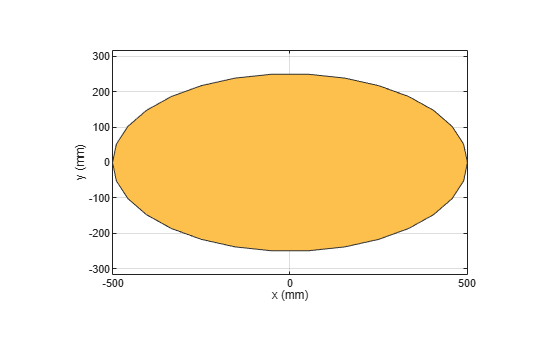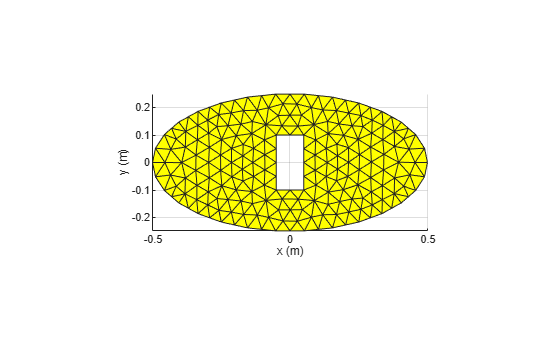antenna.Ellipse

Create ellipse centered at origin on X-Y plane

Description

Use the antenna.Ellipse object to create an ellipse centered at the origin on the X-Y plane.

Creation

Description

example

ellipse = antenna.Ellipse creates an ellipse centered at the origin on the X-Y plane.

example

ellipse = antenna.Ellipse(Name,Value) sets properties using one or more name-value pair arguments. For example, ellipse = antenna.Ellipse('MajorAxis',2,'Minoraxis',0.800) creates an ellipse with a longest diameter of 2 m and smallest diameter of 0.8 m. Enclose each property name in quotes.

Properties

expand all

Name of ellipse, specified as a character vector.

Example: 'Name','ellipse1'

Example: ellipse.Name= 'ellipse1'

Data Types: char | string

Cartesian coordinates of center of ellipse, specified as a two-element vector with each element measured in meters.

Example: 'Center',[0.006 0.006]

Example: Ellipse.Center= [0.006 0.006]

Data Types: double

Major axis of ellipse, specified as a scalar in meters.

Example: 'MajorAxis',1

Example: ellipse.MajorAxis= 2

Data Types: double

Minor axis of the ellipse, specified as a scalar in meters.

Example: 'MinorAxis',0.9

Example: ellipse.MinorAxis= 0.8

Data Types: double

Number of discretization points on circumference, specified as a scalar.

Example: 'NumPoints',28

Example: ellipse.NumPoints= 60

Data Types: double

Object Functions

 add Boolean unite operation on two shapes subtract Boolean subtraction operation on two shapes intersect Boolean intersection operation on two shapes plus Shape1 + Shape2 minus Shape1 - Shape2 and Shape1 & Shape2 area Calculate area of shape in square meters show Display antenna or array structure; display shape as filled patch plot Plot boundary of shape mesh Mesh properties of metal or dielectric antenna or array structure rotate Rotate shape about axis and angle rotateX Rotate shape about x-axis and angle rotateY Rotate shape about y-axis and angle rotateZ Rotate shape about z-axis and angle translate Move shape to new location scale Change the size of the shape by a fixed amount removeHoles Remove holes from shape removeSlivers Remove sliver outliers from boundary of shape

Examples

collapse all

Create ellipse using antenna.Ellipse.

e1 = antenna.Ellipse
e1 =
Ellipse with properties:

Name: 'myEllipse'
Center: [0 0]
MajorAxis: 1
MinorAxis: 0.5000
NumPoints: 30

View the antenna.Ellipse object using the show function.

show(e1)Create an ellipse with major axis of 2 m and a minor axis of 0.8 m.

e2 = antenna.Ellipse('MajorAxis',2,'MinorAxis',0.8)
e2 =
Ellipse with properties:

Name: 'myEllipse'
Center: [0 0]
MajorAxis: 2
MinorAxis: 0.8000
NumPoints: 30

Create a mesh with a Maximum edge Length of 20 cm.

mesh(e2,'MaxEdgeLength',2e-1)Create an ellipse with default properties.

e3 = antenna.Ellipse;

Create a rectangle with a length of 0.1 m and width of 0.2 m.

r = antenna.Rectangle('Length',0.1,'Width',0.2);

Subtract the two shapes using the minus operator.

s = e3-r;

Mesh the subtracted shape with a maximum edge length of 1 m.

mesh(s,1)